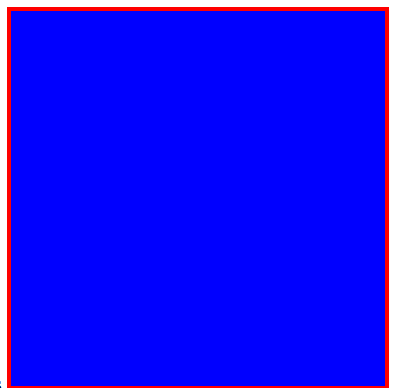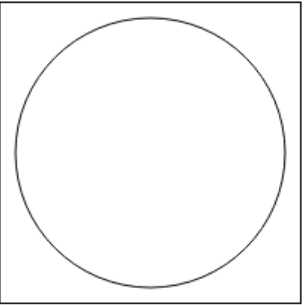# How to Draw Graphics using Canvas in HTML5 ?

In this article, we will draw graphics by using the canvas element in the document. This tag in HTML is used to draw graphics on a web page using JavaScript. It can be used to draw paths, boxes, texts, gradient, and adding images. By default, it does not contain borders and text.

Note: This tag is new in HTML5.

Syntax:

`<canvas id = "script"> Contents... </canvas>`

Example 1:

 ` ` `<``html``> ` ` `  `<``head``> ` `    ``<``meta` `charset``=``"utf-8"``> ` `    ``<``title``> ` `        ``How to Draw Graphics using ` `        ``Canvas in HTML5 ? ` `    `` ` `     `  `    ``<``style``> ` `        ``#FirstCanvas { ` `            ``width: 300px; ` `            ``height: 300px; ` `            ``border: 3px solid red; ` `            ``background-color: blue; ` `        ``} ` `    `` ` ` ` ` `  `<``body``> ` `    ``<``canvas` `id``=``"FirstCanvas"``> ` ` ` ` `  ` `

Output:Example 2:

 ` ` `<``html``> ` ` `  `<``head``> ` `    ``<``title``> ` `        ``How to Draw Graphics  ` `        ``using Canvas in HTML5? ` `    `` ` ` ` ` `  `<``body``> ` `    ``<``canvas` `id``=``"geeks"` `height``=``"200"` `            ``width``=``"200"` `style``= ` `            ``"border:1px solid black"``> ` `    `` ` ` `  `    ``<``script``> ` `        ``var c = document.getElementById("geeks"); ` `        ``var cx = c.getContext("2d"); ` `        ``cx.beginPath(); ` `        ``cx.arc(100, 100, 90, 0, 2 * Math.PI); ` `        ``cx.stroke();  ` `    `` ` ` ` ` `  ` `

Output:Supported Browsers: